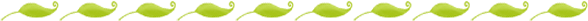# Why is Place Value Important?

When I was a child learning mathematics, the emphasis was on memorizing facts and algorithms. When I was taught place value the lesson went something like this: a number in this location is in the tens place, a number in this location is in the ones place, remember that, there will be a test.

I never understood the purpose of place value, and it was only when I became a teacher myself that I came to understand the invaluable tool that it can be when solving mathematical problems and in understanding our number system!

It is not an understanding of the labels “tens place” and “ones place” that are important when teaching place value, it is the concept of exchange that they represent. In our number system there are only 9 numerals, 1, 2, 3, 4, 5, 6, 7, 8, 9. In order to represent any quantity larger than these numerals we “reset” one column and start over in the next, creating the number “10”. Our number system is a base ten system because that is the amount at which we run out of numerals and must start in another column.

Young children often confuse large numbers like 19 and 91; this is because they do not have an understanding of the exchange that has taken place to create the numerals. 19 is really 10 + 9 and 91 is really 90 + 1. Once a child understands what the 1 and the 9 mean, depending on where they are located, they will have no trouble distinguishing between large numbers. Understanding what a numeral means in context will also serve children well when they add and subtract digits with more than one numeral. For example, if a child needs to add 31 and 42 and knows that they are really adding 30 + 40 + 1 + 2, the solution becomes more apparent. If a child needs to add 48+32= and they understand that they are adding 40+8 and 30+2 they can "borrow" the 2 from 32 and add it to 48, making the problem 50+30, a much simpler problem to solve!

These place value lessons are designed to increase a childʼs understanding of the number system and how context determines the meaning of a numeral.

In order for children to be successful in these lessons they must first understand the concept of skip counting and be able to count by tens. The lessons are organized in order of difficulty, progressing from the earliest concepts of place value to those that develop later. Ideas have been included at the end of some lessons of ways in which you can extend a lesson if your students need more practice on a particular concept. I hope they will be helpful in your classroom and will help your children not only be able to improve
in mathematics, but to think more like mathematicians.

----Submitted by Lyndsey